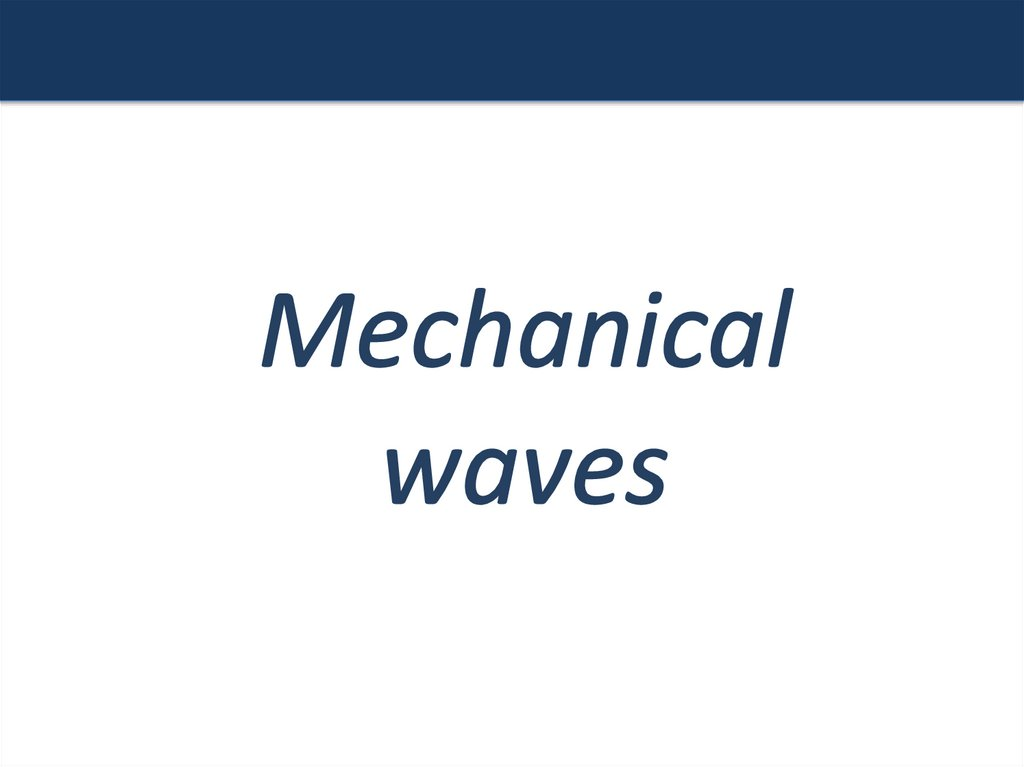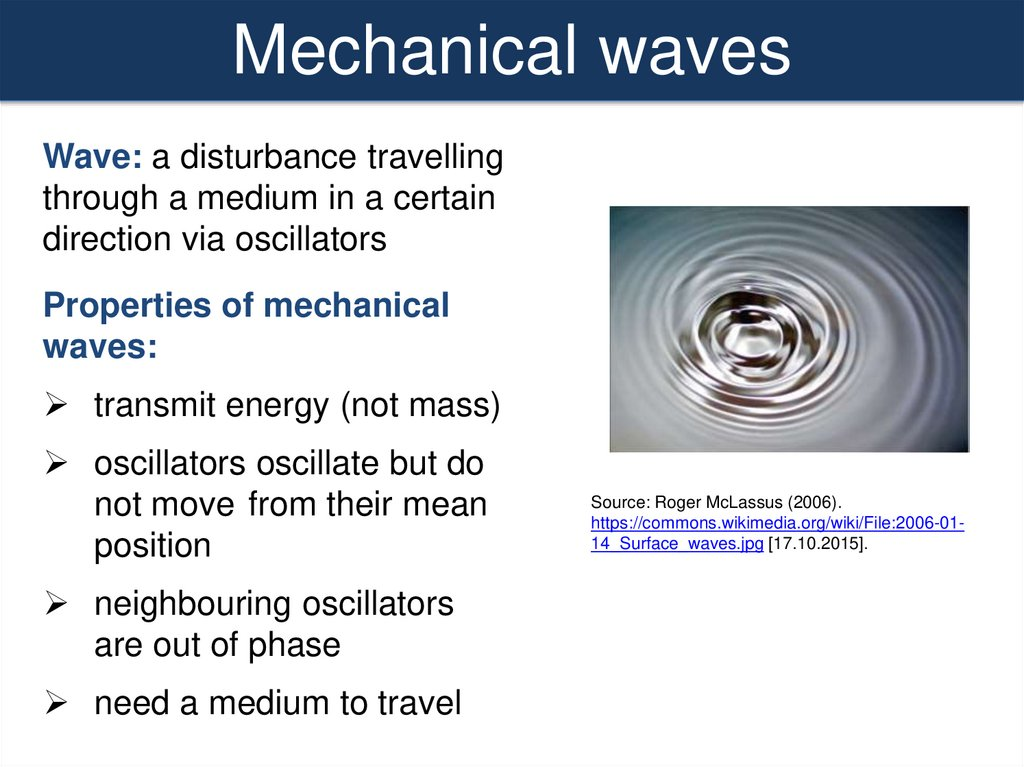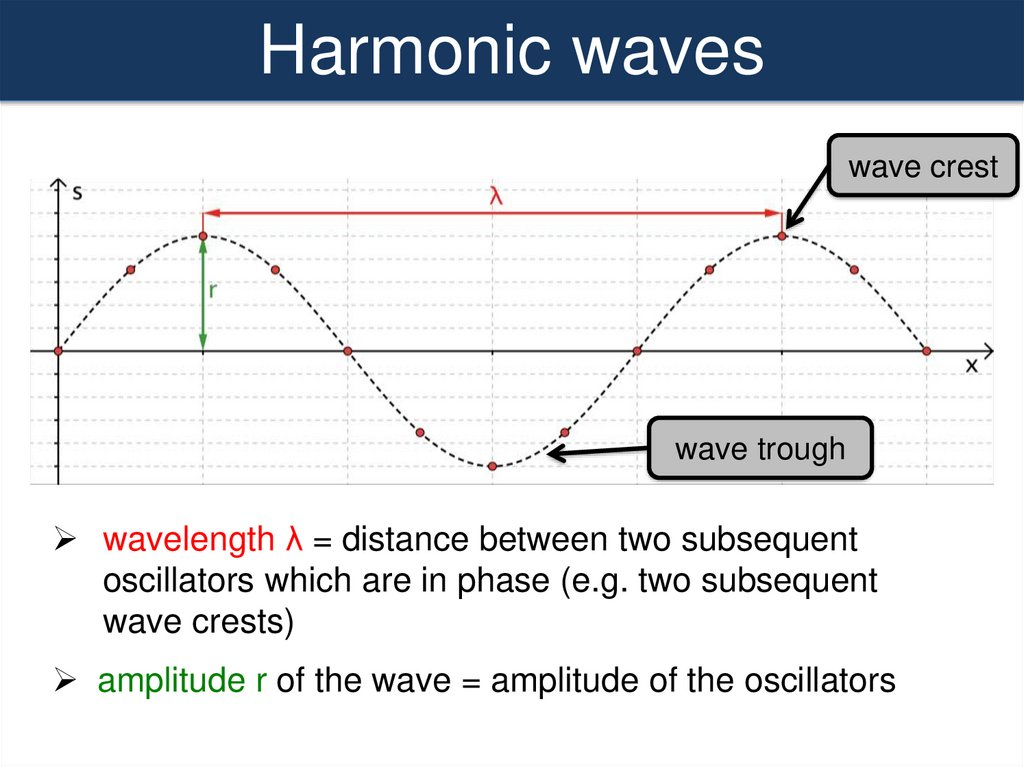# Mechanical waves

## 2.

Mechanical waves
Wave: a disturbance travelling
through a medium in a certain
direction via oscillators
Properties of mechanical
waves:
transmit energy (not mass)
oscillators oscillate but do
not move from their mean
position
neighbouring oscillators
are out of phase
need a medium to travel
Source: Roger McLassus (2006).
https://commons.wikimedia.org/wiki/File:2006-0114_Surface_waves.jpg [17.10.2015].

## 3.

Harmonic waves
wave crest
wave trough
wavelength λ = distance between two subsequent
oscillators which are in phase (e.g. two subsequent
wave crests)
amplitude r of the wave = amplitude of the oscillators

## 4.

Harmonic waves
all oscillators are harmonic oscillators
all oscillators have the same period, frequency and
amplitude (without damping)
frequency f of the wave = frequency of the oscillators
speed c of the wave:
c
x
c
or c f
t T
T

## 5.

Using sine curves to analyse waves (pg. 129)
displacement-distance graph of
wave motion:
displacement – time graph of an
oscillator propagating a wave:
snapshot of positions of all
oscillators that transmit a wave at
one instant in time
shows amplitude (A) and
wavelength (λ) of the wave
shows amplitude (A) and period (T)
of all individual harmonic oscillators
of the wave
If oscillators are harmonic:
f wave = f oscillator

## 6.

Wavefronts (pg.123)
imaginary lines which join identical points (oscillators)
of several waves which are in phase (in the same phase
of their motion)
eg. all crests or troughs
show in which way a wave is moving

## 7.

Energy and intensity (pg. 126-127)
Energy
the energy of the wave is the sum of energies of the
single oscillators
proportional to the amplitude (r) squared
proportional to the frequency squared
m r 2 2
E
2
Intensity (unit: W/m2)
total power transmitted
P
intensity
or =
total area through which the waves pass
A
P
4πr 2

## 8.

Transverse waves
Types of mechanical waves
oscillators
wave direction
The direction of vibration of the particles is perpendicular to the
direction of propagation of the wave.

## 9.

Longitudinal waves
wave direction
The direction of vibration of the particles is parallel to the
direction of propagation of the wave.
Pattern of compression (particle spacing decreases) and
rarefaction (particle spacing increases)

## 10.

Using sine curves to analyse longitudinal waves
1) pressure-distance graphs: show instantaneous positions of
pressure peaks (maximum compressions) and troughs
(maximum rarefactions) at a point in time
http://hyperphysics.phy-astr.gsu.edu/hbase/Sound/tralon.html

## 11.

Using sine curves to analyse longitudinal waves
2) displacement (from equilibrium) – distance graph:
• shows instantaneous positions of oscillators relative to their
equilibrium position at a point in time
https://funwaves.wikispaces.com/5.+Longitudinal+wave